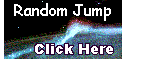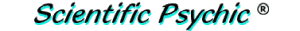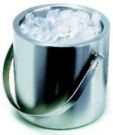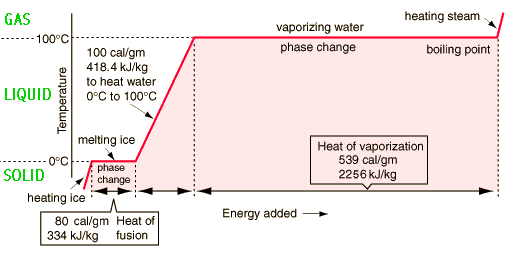Index#The Cold DrinkAt a bar, there is a bucket containing ice, some of which has melted. A bartender gets an ice cube weighing 20 grams from the ice bucket and puts it into an insulated cup containing 100 grams of water at 20 degrees Celsius. Will the ice cube melt completely? What will be the final temperature of the water in the cup?

First of all, you have to know that the ice in the bucket has a temperature of 0 degrees Celsius. How do we know this? The Celsius temperature scale is defined to be 0°C when ice is in thermal equilibrium with water, and 100°C when water is in equilibrium with steam. An ice bucket does not provide extra cooling, it just maintains ice at its equilibrium melting point temperature.

The Specific Heat (Sh) of a substance is the amount of heat required to change the temperature of 1 gram of the substance by one degree Celsius. By definition, the Specific Heat of water is 1.0, and this amount of heat is called a calorie. A kilocalorie is 1000 calories, also called a "Calorie" with upper case. The Calories in nutrition labels are kilocalories.

Latent heat is the heat required to change the state of a substance from solid to liquid, or from liquid to gas. Water is in thermal equilibrium with ice when it freezes at the same rate that the ice melts. The heat for melting or freezing water, also called the Heat of Fusion (Hf), is 80 calories per gram at 0°C, which is the equilibrium temperature. In other words, it is necessary to add 80 calories of energy to melt one gram of ice at 0°C. Conversely, it is necessary to remove 80 calories of energy to freeze one gram of water at 0°C. The heat for boiling or condensing water at 100°C, called the Heat of Vaporization, is 539 calories per gram, although this is not relevant to our problem. The following graph summarizes the effect of energy on the temperature of water in its solid, liquid, and gaseous phases.The problem can be solved by breaking it into smaller pieces. The amount of energy needed to melt the ice is Wi×Hf, where Wi is the weight of the ice. In addition, the 20 grams of water obtained from the ice must be heated from 0°C, which is the temperature of the ice, to the final temperature Tf.

Where does the energy for melting the ice come from? Since the cup is insulated, the heat has to come from the 100 grams of water at 20°C. The amount of heat required to melt the ice and to increase the temperature of the resulting water to the final temperature is equal to the heat lost by the 100 grams of water. This can be expressed by an equation:

Wi×Hf + Wi×Sh×(Tf - 0°C) = Ww×Sh×(Twi - Tf)
Where
Wi = weight of ice = 20g
Hf = Heat of fusion = 80 calories/gram
Ww = weight of water = 100g
Tf = final temperature,
Twi = initial water temperature = 20°C
Sh = Specific Heat for water = 1.0 calories per gram per °C

Substituting the values we get:
20×80 + 20×1×(Tf - 0°C) = 100×1×(20°C - Tf)
1600 + 20Tf = 2000 - 100Tf
120Tf = 400
Tf = 400/120 = 3.3°C

The final temperature is 3.3°C. If we had had more ice or less water, there would not have been enough energy in the water to melt all the ice. Once the temperature of the water reaches 0°C, no more ice can melt.

How much ice could be melted by 100 g of water at 20°C? Using the equation above, where the final temperature is 0°C and the weight of the ice is unknown, we have:

Wi×80 = 100×1×20

The maximum amount of ice that could be melted is:
Wi = 2000/80 = 25 grams

If the amount of ice exceeds 25 grams, the remainder will just float in 125 grams of water at 0°C.

The original version of this problem did not state that the ice in the bucket had been melting, and a reader thought that it was incorrect to assume that the temperature of the ice in the bucket was 0°C. He said:

A cube of ice can be chilled down well below 0 degrees Celsius. If one were to slowly cool a quantity of water until it was completely frozen, I agree that any ice formed could be assumed to have a temperature of 0 degrees Celsius, assuming some liquid water remained. Not so for melting ice. Assuming the temperature outside the bucket is higher than the temperature of the ice cubes (I live in a mountain town where temperatures regularly dip below -30 degrees Fahrenheit, and we put our beers in a cooler to prevent freezing during hockey games), the ice temperature will rise, and the surface temperature of any cube will be generally higher than that of the center. If no melting has yet occurred in the bucket, the surface temperature of the cubes must still be below 0 degrees Celsius. If some melting has already occurred, then the surface temperature must be at exactly 0 degrees Celsius, while the center of each cube would remain lower. True, if the cubes were warmed very, very slowly, the center temperature of the melting cubes could approach 0 degrees Celsius just as the cubes began to melt, but in any real-world scenario this is very unlikely. In any event, the solution as given likely underestimates the energy needed to melt the ice, as it almost certainly would require at least some energy simply to bring the ice up to 0 degrees Celsius.

Another reader thought that a time should have been specified for measuring the temperature since a cup is defined to be an open container, and the contents of the cup will eventually come to thermal equilibrium with the environment.

You are missing at least one vital piece of information in the above referenced questions. You are missing a length of time to make the determination of the final water temperature. While you do state that you are using a insulated cup, a cup as defined by Merriam-Webster is an open, usually bowl-shaped drinking vessel. This would mean that it is open to the environment. Therefore, without a given length of time to make the measurement, the temperature of the water in the cup will continue to go up until it reaches the environment temperature. If you do not wish to state a given length of time to make the measurement, then you need to state the environment temperature.

The problem should have said "an insulated cup with an insulated cap", better known as a calorimeter.

The Ninth Edition of the Merk Index lists the heat of fusion of water as 1.436 kcal/mole and the heat of vaporization as 9.717 kcal/mole. Since the molecular weight of water is 18.016 g/mole, Hf = 79.71 cal/g, and Hv = 539.35 cal/g.